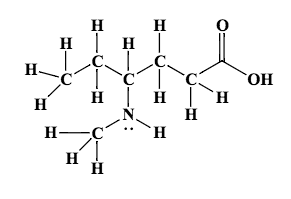# Problem: Convert the following structural formula into the carbon skeleton formula, otherwise known as a kekulé structure.

###### FREE Expert Solution
88% (486 ratings)
###### Problem Details

Convert the following structural formula into the carbon skeleton formula, otherwise known as a kekulé structure.What scientific concept do you need to know in order to solve this problem?

Our tutors have indicated that to solve this problem you will need to apply the Structural Formula concept. You can view video lessons to learn Structural Formula. Or if you need more Structural Formula practice, you can also practice Structural Formula practice problems.

What is the difficulty of this problem?

Our tutors rated the difficulty ofConvert the following structural formula into the carbon ske...as medium difficulty.

How long does this problem take to solve?

Our expert Chemistry tutor, Jules took 2 minutes and 38 seconds to solve this problem. You can follow their steps in the video explanation above.

What professor is this problem relevant for?

Based on our data, we think this problem is relevant for Professor Ratliff's class at USF.